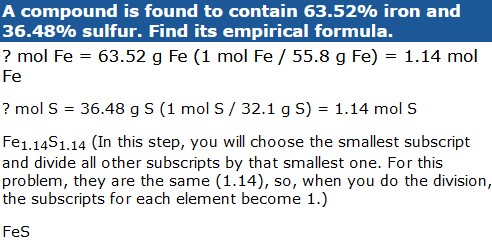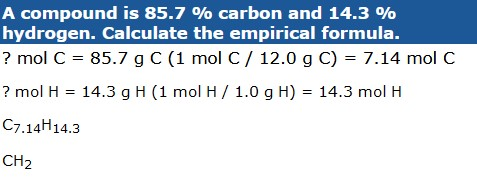# Percent Composition and Empirical Formula Practice

## Self-Assessment and Practice

% Composition

1. Calculate the percent composition of HCl.

HINT

When you are only given a compound formula, start by calculating the molar mass of the compound.

1.0 g + 35.5 g = 36.5 g (molar masses from the periodic table)
H (1.0 g/36.5 g) x100% = 2.7% H
Cl (35.5 g/36.5 g) x 100% = 97.3% Cl

2. Calculate the percent composition of K2CO3

2(39.1 g) + 12.0 g +3(16.0 g) = 138.2 g
%K = (78.2g/138.2 g) x 100% = 56.6% K
%C = (12.0 g/138.2 g) x 100% = 8.68% C
%O = (48.0 g/138.2 g) x 100% = 34.7% O

3. Calculate the percent composition for Mg(OH)2.

24.3 g + 2(16.0 g) + 2(1.0 g) = 58.3 g
%Mg = (24.3g/58.3g) x 100% = 42.7%
%O = (32.0g/58.3g) x 100% = 54.9%
%H = (2.0g/58.3g) x 100% = 3.4%

4. Calculate the percent composition for potassium sulfate.

HINT

You must first be certain that you have the correct formula for potassium sulfate. Refer to an ionic charges chart.

K2SO4
2(39.1 g) + 32.1 g + 4(16.0 g) = 174.3 g
%K = (78.2 g/174.3 g) x 100% = 44.9%
%S = (32.1 g/174.3 g) x 100% = 18.4%
%O = (64.0 g/174.3 g) x 100% = 36.7%

Empirical Formulas

Work each of the following problems.

1. A compound is found to contain 63.52% iron and 36.48% sulfur. Find its empirical formula.

HINT

When you are given the percentage composition of a compound and asked to find the empirical formula, just assume that you have 100g of the substance. This allows you to turn your % into grams. Out of 100 g of this compound of iron and sulfur, 63.52g are iron and 36.48g are sulfur.2. In the laboratory, a sample is found to contain 1.05 grams of nickel and 0.29 grams oxygen. Determine the empirical formula.3. What is the empirical formula for a compound if a 2.50 g sample contains 0.900 g of calcium and 1.60 g of chlorine?